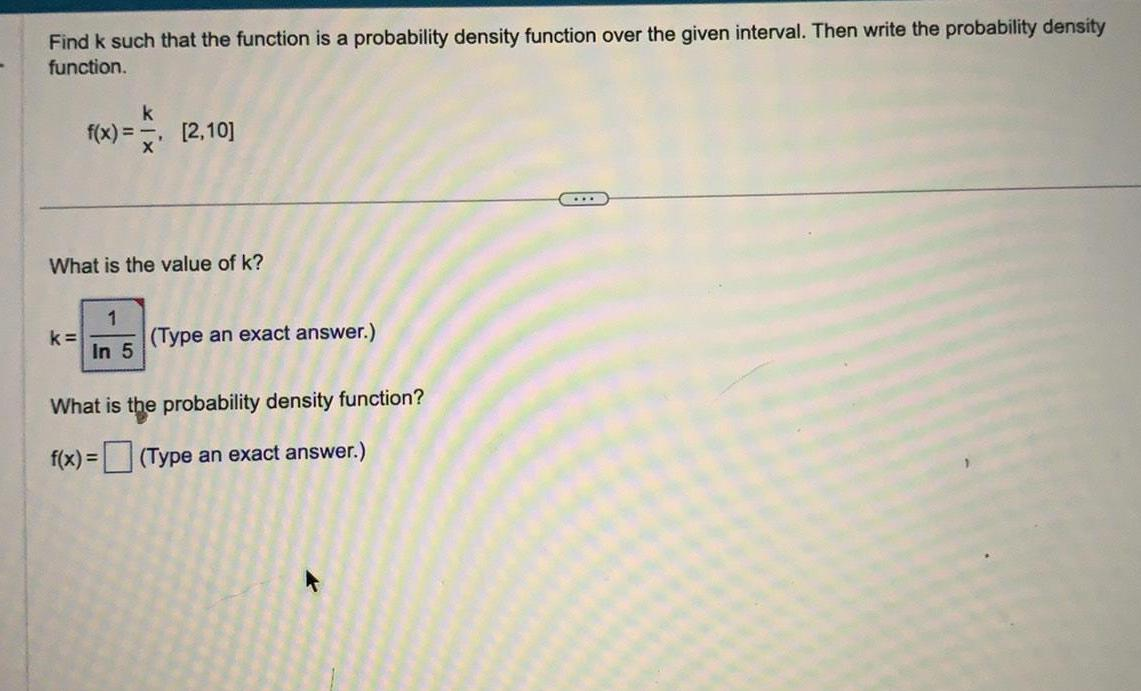Question:

# Find k such that the function is a probability density

Last updated: 7/22/2022Find k such that the function is a probability density function over the given interval. Then write the probability density function. f(x) =k/ x [2,10] What is the value of k? k= (Type an exact answer.) What is the probability density function? f(x) = (Type an exact answer.)